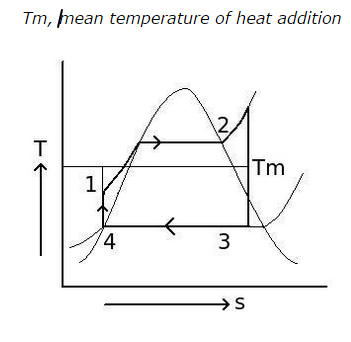# Comparison of Rankine and Carnot Cycles - MCQs with Answers

## Comparison of Rankine and Carnot Cycles - MCQs with Answers

Q1. How can we differentiate Rankine cycle from Carnot cycle?

a. Heat addition process of Rankine cycle is reversible isothermal whereas heat addition process of Carnot cycle is reversible isobaric

b. Heat addition process of Rankine cycle is reversible isobaric whereas heat addition process of Carnot cycle is reversible isothermal

c. Heat addition process of Rankine cycle is reversible isentropic whereas heat addition process of Carnot cycle is reversible isothermal

d. both cycles are identical except the working fluid used

ANSWER: b. Heat addition process of Rankine cycle is reversible isobaric whereas heat addition process of Carnot cycle is reversible isothermal

Q2. What is the relation between efficiencies of Rankine cycle () and Carnot cycle for the same pressure ratio?

a. (ηRankine) = (ηCarnot)
b. (ηRankine) > (ηCarnot )
c. (ηRankine) < (ηCarnot )
d. none of the above

ANSWER: c. (ηRankine) < (ηCarnot )

Q3. If Tm be the mean temperature of heat addition in Rankine cycle as shown in diagram,
what will the formula for efficiency of Rankine cycle?a. (ηRankine) = ( T3 / Tm)
b. (ηRankine) = 1 – (T3 / Tm)
c. (ηRankine) = 1 – (T2 / Tm)
d. (ηRankine) = (T2 / Tm)

ANSWER: b. (ηRankine) = 1 – (T3 / Tm)

Q4. The maximum efficiency of Rankine cycle (ηRankine) is the function of

a. the mean temperature of heat addition (Tm) only
b. the mean temperature of heat addition (Tm) and temperature of steam at the exit of the turbine
c. the mean temperature of heat addition (Tm) and temperature of steam at the entry of the turbine
d. the mean temperature of heat addition (Tm) and temperature of steam at exit of the condenser

Q5. What is the effect of superheated steam on efficiency of Rankine cycle?

a. efficiency of Rankine cycle decreases with increase in superheat of the steam
b. efficiency of Rankine cycle increases with increase in superheat of the steam
c. efficiency of Rankine cycle is not affected by change in superheat of the steam
d. none of the above

ANSWER: b. efficiency of Rankine cycle increases with increase in superheat of the steam

Q6. What is the effect of increase in pressure at which heat is added on the pump work in the Rankine cycle?

a. the pump work increases with increase in pressure of heat addition
b. the pump work decreases with increase in pressure of heat addition
c. the pump work does not change with increase in pressure of heat addition
d. the pump work either increases or decreases with increase in pressure of heat addition

ANSWER: a. the pump work increases with increase in pressure of heat addition

Q7. When the pressure at which heat is added in Rankine cycle increases, the moisture content at the turbine exhaust

a. increases
b. decreases
c. remains same
d. cannot say

Q8. What is the condition for increase the chances of corrosion of blades of turbine?

a. decrease in the pressure difference between which the Rankine cycle operates
b. increase in the pressure difference between which the Rankine cycle operates
c. both a. and b.
d. none of the above

ANSWER: b. increase in the pressure difference between which the Rankine cycle operates

Q9. What is the maximum content of moisture allowed at the turbine exhaust in the steam power plant?

a. 50 %
b. 60 %
c. 30 %
d. 15 %